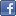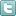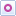EE Times-India > Controls/MCUs

Controls/MCUs# Guide to field-oriented control: Step 2

Posted: 27 Mar 2015Print Version

Keywords:Field-oriented control  FOC  AC motor  DC motors  torque

In the first part of this series, we found out that field-oriented control (FOC) of an AC motor is not that dissimilar to current control of a brush DC motor (figure 1). We partitioned the process into four discrete steps and covered the first step, measuring the motor's current. Now let's discuss the second and most important step, which is the heart of the entire process.

Compare the measured currents to the desired currents, and generate error signals. While this comparison step is very straightforward for a brush DC motor, there are a few more operations required for an AC motor.Figure 1: Current control of a brush DC motor.

But please, don't get lost in the minutia. Remember the end goal of what we are trying to do here.

First, let's simplify our three-phase system to an equivalent two-phase system. One way to visualise this conversion is to realise that we have three axes of current, which combine to create a current vector in two-dimensional space. But for two dimensions we only need two axes to define any vector.

This issue was first addressed by Edith Clarke during her career at General Electric (1922-46). (Incidentally, she was the first woman to receive a Master's Degree in Electrical Engineering from MIT, and also the first female professor of electrical engineering in the United States at the University of Texas, Austin.) Her famous set of equations convert a three-phase system into an equivalent two-phase system without any loss of information.Equation 1: Amplitude invariant form of the Clarke transform.

This set of equations is referred to mathematically as the αβγ transform, but it is more widely known by power engineers as the Clarke transform.

Another way to think of the Clarke transform is that it takes three coils evenly spaced 120° apart, and turns them into two equivalent coils, one at 0° and one at 90°. The equations shown are amplitude invariant, which means the outputs are scaled so that the resulting two-phase waveforms have the same amplitude as the original three-phase waveforms. The last equation accounts for any common-mode currents. But for three-phase motors, ia(t) + ib(t) + ic(t) = 0, so the last equation is often omitted in FOC current calculations. This results in the net current vector being resolved into x and y components on a standard rectangular coordinate system, as shown in figure 2.Figure 2: Graphical representation of the Clarke transform.

Using the Clarke transform, we can design an FOC system as shown in figure 3. But this design is rarely used. While the system in figure 3 will work okay at low speeds, at high speeds the bandwidth limitation of the P-I controllers will make it harder to follow the reference current waveforms.Figure 3: Stationary frame current regulator FOC system.

 Related Articles Editor's Choice
Comment on "Guide to field-oriented control: Ste..."

Top Ranked Articles

WebinarsVisit Asia Webinars to learn about the latest in technology and get practical design tips.

Search EE Times India
Services

Go to topConnect on FacebookFollow us on TwitterFollow us on Orkut
﻿